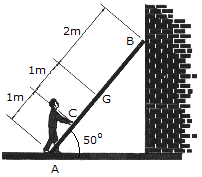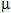# Engineering Mechanics - Friction - Discussion

### Discussion :: Friction - General Questions (Q.No.5)

5.A 17-kg ladder has a center of mass at G. If the coefficients of friction at A and B areA = 0.3 andB = 0.2, respectively, determine the smallest horizontal force that the man must exert of the ladder at point C in order to push the ladder forward.

 [A]. F = 120.2 N [B]. F = 288 N [C]. F = 166.8 N [D]. F = 204 N

Explanation:

No answer description available for this question.

 Rathish Kumar Singh. said: (Feb 11, 2012) Apply the frictional forces at points A and B, the normal reactions at A and B. Write the conditions of equilibirium. But take the moments about the centre of gravity of ladder, you will get afterwards the required force P.

 Rajni Mallick said: (Oct 8, 2014) My Question 1. A block of mass m rests in a V- shaped channel as shown in fig (a) & (b) determine what horizontal force P must be applied for the motion to impend.

 Arunkumar said: (Mar 13, 2015) Shall any one explain in detail?